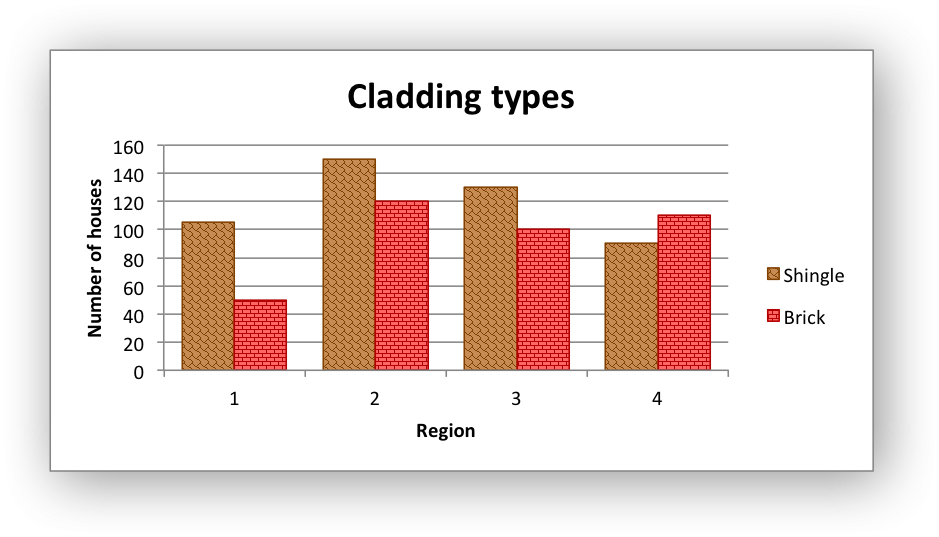libxlsxwriter
chart_pattern.c

An example of creating a simple chart with different patterns./*
* An example of a simple Excel chart with patterns using the libxlsxwriter
* library.
*
* Copyright 2014-2021, John McNamara, jmcnamara@cpan.org
*
*/
#include "xlsxwriter.h"
/* Create a worksheet with a chart. */
int main() {
lxw_workbook *workbook = workbook_new("chart_pattern.xlsx");
lxw_chart *chart;
/* Add a bold format to use to highlight the header cells. */
/* Write some data for the chart. */
worksheet_write_string(worksheet, 0, 0, "Shingle", bold);
worksheet_write_number(worksheet, 1, 0, 105, NULL);
worksheet_write_number(worksheet, 2, 0, 150, NULL);
worksheet_write_number(worksheet, 3, 0, 130, NULL);
worksheet_write_number(worksheet, 4, 0, 90, NULL);
worksheet_write_string(worksheet, 0, 1, "Brick", bold);
worksheet_write_number(worksheet, 1, 1, 50, NULL);
worksheet_write_number(worksheet, 2, 1, 120, NULL);
worksheet_write_number(worksheet, 3, 1, 100, NULL);
worksheet_write_number(worksheet, 4, 1, 110, NULL);
/* Create a chart object. */
/* Configure the chart. */
lxw_chart_series *series1 = chart_add_series(chart, NULL, "Sheet1!\$A\$2:\$A\$5");
lxw_chart_series *series2 = chart_add_series(chart, NULL, "Sheet1!\$B\$2:\$B\$5");
chart_series_set_name(series1, "=Sheet1!\$A\$1");
chart_series_set_name(series2, "=Sheet1!\$B\$1");
chart_axis_set_name(chart->x_axis, "Region");
chart_axis_set_name(chart->y_axis, "Number of houses");
/* Configure an add the chart series patterns. */
.fg_color = 0x804000,
.bg_color = 0XC68C53};
.fg_color = 0XB30000,
.bg_color = 0XFF6666};
chart_series_set_pattern(series1, &pattern1);
chart_series_set_pattern(series2, &pattern2);
/* Configure and set the chart series borders. */
lxw_chart_line line1 = {.color = 0x804000};
lxw_chart_line line2 = {.color = 0xb30000};
chart_series_set_line(series1, &line1);
chart_series_set_line(series2, &line2);
/* Widen the gap between the series/categories. */
/* Insert the chart into the worksheet. */
worksheet_insert_chart(worksheet, CELL("D2"), chart);
return workbook_close(workbook);
}
lxw_chart_pattern
Struct to represent a chart pattern.
Definition: chart.h:681
workbook_close
lxw_error workbook_close(lxw_workbook *workbook)
Close the Workbook object and write the XLSX file.
chart_series_set_pattern
void chart_series_set_pattern(lxw_chart_series *series, lxw_chart_pattern *pattern)
Set the pattern properties for a chart series.
chart_series_set_name
void chart_series_set_name(lxw_chart_series *series, const char *name)
Set the name of a chart series range.
chart_axis_set_name
void chart_axis_set_name(lxw_chart_axis *axis, const char *name)
Set the name caption of the an axis.
lxw_chart_series * chart_add_series(lxw_chart *chart, const char *categories, const char *values)
Add a data series to a chart.
LXW_CHART_PATTERN_HORIZONTAL_BRICK
@ LXW_CHART_PATTERN_HORIZONTAL_BRICK
Definition: chart.h:379
workbook_new
lxw_workbook * workbook_new(const char *filename)
Create a new workbook object.
format_set_bold
void format_set_bold(lxw_format *format)
Turn on bold for the format font.
lxw_chart_line
Struct to represent a chart line.
Definition: chart.h:639
lxw_worksheet
Struct to represent an Excel worksheet.
Definition: worksheet.h:2107
lxw_format
Struct to represent the formatting properties of an Excel format.
Definition: format.h:358
lxw_chart
Struct to represent an Excel chart.
Definition: chart.h:1090
worksheet_insert_chart
lxw_error worksheet_insert_chart(lxw_worksheet *worksheet, lxw_row_t row, lxw_col_t col, lxw_chart *chart)
Insert a chart object into a worksheet.
lxw_chart_series
Struct to represent an Excel chart data series.
Definition: chart.h:960
chart_set_series_gap
void chart_set_series_gap(lxw_chart *chart, uint16_t gap)
Set the gap between series in a Bar/Column chart.
lxw_chart::y_axis
lxw_chart_axis * y_axis
Definition: chart.h:1111
lxw_chart_line::color
lxw_color_t color
Definition: chart.h:642
lxw_workbook
Struct to represent an Excel workbook.
Definition: workbook.h:292
lxw_chart::x_axis
lxw_chart_axis * x_axis
Definition: chart.h:1105
LXW_CHART_PATTERN_SHINGLE
@ LXW_CHART_PATTERN_SHINGLE
Definition: chart.h:397
chart_title_set_name
void chart_title_set_name(lxw_chart *chart, const char *name)
Set the title of the chart.
chart_series_set_line
void chart_series_set_line(lxw_chart_series *series, lxw_chart_line *line)
Set the line properties for a chart series.
worksheet_write_string
lxw_error worksheet_write_string(lxw_worksheet *worksheet, lxw_row_t row, lxw_col_t col, const char *string, lxw_format *format)
Write a string to a worksheet cell.
worksheet_write_number
lxw_error worksheet_write_number(lxw_worksheet *worksheet, lxw_row_t row, lxw_col_t col, double number, lxw_format *format)
Write a number to a worksheet cell.
lxw_chart_pattern::type
uint8_t type
Definition: chart.h:690
CELL
#define CELL(cell)
Convert an Excel A1 cell string into a (row, col) pair.
Definition: utility.h:45